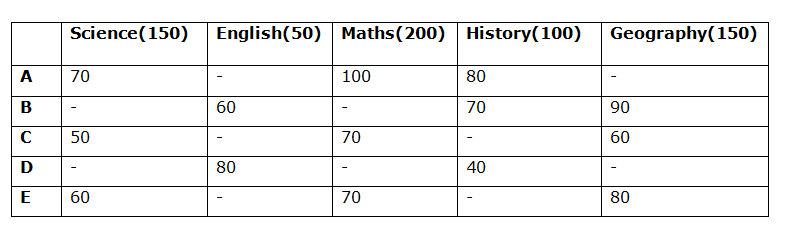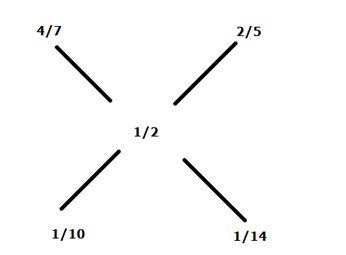# SBI PO Prelims Quantitative Aptitude Questions 2019 (Day-52)

Dear Aspirants, Our IBPS Guide team is providing new series of Quantitative Aptitude Questions for SBI PO 2019 so the aspirants can practice it on a daily basis. These questions are framed by our skilled experts after understanding your needs thoroughly. Aspirants can practice these new series questions daily to familiarize with the exact exam pattern and make your preparation effective.

[WpProQuiz 6408]

### Click Here for SBI PO Pre 2019 High-Quality Mocks Exactly on SBI Standard

Direction (1 – 5): Study the following information carefully and answer the given questions:

The given table shows the percentage of the marks in 5 different students in five different subjects. Maximum marks of subjects – Science, English, Maths, History and Geography is 150, 50, 200, 100 and 150 respectively.1) If the marks of B in Science is 80% of the marks gets by E in Geography and the ratio of the marks of D in Science to the marks of B in History is 4:5, then what is the average mark obtained by all the students in Science?

a) 84.4

b) 78.5

c) 86.8

d) 89.7

e) None of these

2)

If C got 64% as overall percentage in the exam and the ratio of the marks of C in History to the marks of A in English is 3: 1 and the marks of A in English is 33.33% of the marks of E in Science. Find the percentage of C’s marks in English

a) 60%

b) 40%

c) 42%

d) 80%

e) None of these

3) The average mark obtained by all the students in Maths is 140 and the ratio of the marks of B to D in Maths is 5: 6. If the marks of A in Geography is equal to the marks of B in Maths and marks of D in Geography is 150% of the marks of D in English, then find the total marks obtained by all the students in Geography?

a) 485

b) 500

c) 505

d) 515

e) None of these

4) What is ratio of the sum of the marks obtained by A in History and E in Geography together to the sum of the marks obtained by C in Science and C in Geography?

a) 39: 31

b) 40: 33

c) 41: 32

d) 42: 29

e) None of these

5) Total marks obtained by all the students in English is 185 and the marks of E in English is 3/23 of the total marks obtained by B, C and D in Geography and the marks of C in English is 50% of the marks of A in History. What is the ratio of the marks of A, C and E in English?

a) 4: 6: 11

b) 6: 8: 9

c) 14: 11: 25

d) 13: 11: 7

e) None of these

6) A person lent out two equal amounts. One part is simple interest at 12% per annum for 8 years and another part is compound interest at 10% per annum for three years. If the total interest amount is 6196.8, find the amount is lent out each case

a) Rs.4000

b) Rs.4200

c) Rs.4600

d) Rs.4800

e) None of these

7) Jar A contains the mixture of milk and water in the ratio of 3: 2 and the Jar B contains the mixture of milk and water in the ratio of 3: 4. The mixture of both the Jars be mixed obtain the new mixture in Jar C, what is the ratio of mixture in new Jar C contains 50% of milk and 50% of water?

a) 4: 5

b) 6: 7

c) 5: 7

d) 7: 4

e) None of these

8) A train crosses the 300m long bridge in 54 seconds and also crosses the pole in 36 seconds. The same train crosses another 400m long train in 36 seconds. If both the trains are running in opposite direction, then find the speed of second train? (In kmph)

a) 30 kmph

b) 40 kmph

c) 60 kmph

d) Cannot be determine

e) None of these

9) A, B and C can do the work in 12 days, 24 days and 30 days respectively. They all started working together, after 25% of the work completed C leave the job and rest of the work is completed by A and B together. Find the time taken by A and B to complete the remaining work

a) 6 days

b) 4 days

c) 8 days

d) 10 days

e) None of these

10) A and B started the business with the investment of Rs.6000 and Rs.8000 respectively. After 4 months B withdrew 20% of his initial investment and A added 25% of his initial investment. After one year B received the profit share is Rs.7800, then find the total profit?

a) Rs.15675

b) Rs.17865

c) Rs.16545

d) Rs.12875

e) None of these

Marks of A in science = 70/100 * 150 = 105

Marks of C in science = 150 * 50/100 = 75

Marks of E in science = 60/100 * 150 = 90

Marks of B in science = 80/100 * 80/100 * 150 = 96

Marks of B in history = 70/100 * 100 = 70

Marks of D in science = 4/5 * 70 = 56

Required Average = (105 + 75 + 90 + 96 + 56)/5 = 84.4

Marks of A in English = 33.33/100 * 60/100 * 150 = 30

Marks of C in History = 30 * 3/1 = 90

Marks of C in science = 50/100 * 150 = 75

Marks of C in Maths = 70/100 * 200 = 140

Marks of C in Geography = 60/100*150 = 90

Marks of C in English = x

Total marks of C = 90 + 75 + 140 + 90 + x = 395 + x

Total marks = 150 + 50 + 200 + 100 + 150 = 650

395 + x = 64/100 * 650

395 + x = 416

= > x = 416 – 395 = 21

Percentage of marks of C in English = 21/50 * 100 = 42%

Marks of A in Maths = 200

Marks of C in Maths = 70/100 * 200 = 140

Marks of E in Maths = 70/100 * 200 = 140

Total marks of all the students in Maths = 140 * 5 = 700

Marks of B and D in Maths = 700 – 200 – 140 – 140 = 220

Marks of B in Maths = 5/11 * 220 = 100

Marks of A in Geography = Marks of B in Maths = 100

Marks of D in Geography = 150% of Marks of D in English

= 150/100 * 80/100 * 50 = 60

Marks of B in Geography = 90/100 * 150 = 135

Marks of C in Geography = 60/100 * 150 = 90

Marks of E in Geography = 80/100 * 150 = 120

Total marks in Geography = (100 + 60 + 135 + 90 + 120) = 505

Marks of A in History = 80

Marks of E in Geography = 80/100 * 150 = 120

Marks of C in Science = 50/100 * 150 = 75

Marks of C in Geography = 60/100 * 150 = 90

Required ratio = (80 + 120): (75 + 90)

= 200: 165 = 40: 33

Marks of B in Geography = 90/100 * 150 = 135

Marks of C in Geography = 60/100 * 150 = 90

E in Geography = 80/100 * 150 = 120

Marks of E in English = (135 + 90 + 120) * 3/23 = 45

Marks of A in History = 80

Marks of C in English = 80 * 50/100 = 40

Marks of B in English = 60/100 * 50 = 30

Marks of D in English = 80/100 * 50 = 40

Marks of A in English = 185 – (45 + 40 + 30 + 40) = 30

Required ratio = 30: 40: 45

=6: 8: 9

(x * 12 * 8/100) + x * (1 + 10/100)3 – x = 6196.8

96x/100 + 331x/1000 = 6196.8

1291x = 6196800

= > x = 4800= 7: 5

Required ratio = 5: 7

Length of 1st train = L1

Speed of first train when crosses 300 m long bridge = S1

= (L1 + 300)/54 —– (1)

Speed of first train when crosses a pole = S1 = L1/36 —-(2)

(L1 + 300)/54 = L1/36

(L1 + 300)/3 = L1/2

= > 2 L1 + 600 = 3L1

Length of first train = 600 m

S1 = 600/36 = 50/3 m/s

According to the question,

(L1 + L2)/36 = S1 + S2

(600 + 400)/36 = (50/3 + S2)

1000/36 – 50/3 = S2

= > (1000 – 600)/36 = S2

= > 400/36 = S2

S2 = 400/36 * 18/5 = 40 kmph

LCM (12, 24, 30) = 120

A completes = 10 units per day

B completes = 5 units per day

C completes = 4 units per day

25% of the wok=120 * 25/100 = 30 units

Remaining work = 120 – 30 = 90

Time taken by A and B = 90/15 = 6 days

Profit ratio = (6000 * 4 + (7500 * 8)): (8000 * 4 + (6400 * 8))

= 84000: 83200

=105: 104

Total profit = 209/104 * 7800 = Rs.15675